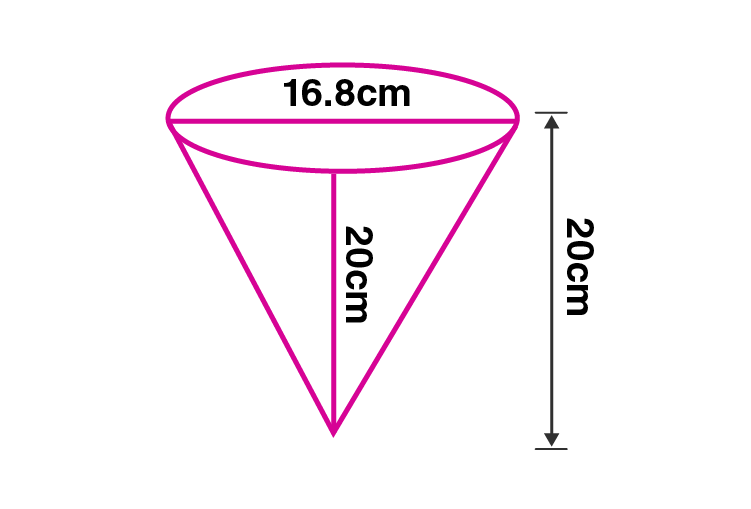Newbie

# A vessel in the form of an inverted cone is filled with water to the brim. Its height is 20 cm and diameter is 16.8 cm. Two equal solid cones are dropped in it so that they are fully submerged. As a result, one-third of the water in the original cone overflows. What is the volume of each of the solid cone submerged?

• 0

An Important Question of class 10 Based on Mensuration Chapter of M.L Aggarwal for ICSE BOARD.
Here is a vessel in the form of an inverted cone is filled with water to the brim. Its height and diameter is given. Two equal solid cones are dropped in it so that they are fully submerged.
What is the volume of each of the solid cone submerged?
This is the Question Number 17, Exercise 17.5 of M.L Aggarwal.

Share

1.Given height of the cone, h = 20 cm

Diameter of the cone = 16.8 cm

Radius of the cone, r = 16.8/2 = 8.4 cm

Volume of water in the vessel = (1/3)r2h

= (1/3)×8.42 ×20

= (1/3)×(22/7)×8.4 ×8.4 ×20

= 1478.4 cm3

One third of volume of water in the vessel = (1/3)× 1478.4

= 492.8 cm3

One third of volume of water in the vessel = Volume of water over flown

Volume of water over flown = volume of two equal solid cones dropped into the vessel.

volume of two equal solid cones dropped into the vessel = 492.8 cm3

Volume of one solid cone dropped into the vessel = 492.8/2 = 246.4 cm3

Hence the volume of each of the solid cone submerged is 246.4 cm3.

• 0